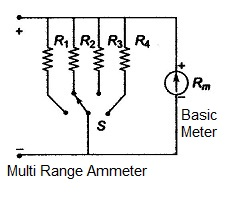# Multi Range Ammeter with Circuit

Multi Range Ammeter:

The same basic moving coil ammeter can be utilized to give full scale deflection for different ranges of measurement. Such an arrangement is shown below with Multi Range Ammeter circuit diagram.Multi Range Ammeter with Individual Shunt Resistance
The circuit under figure (In figure R1 = Rsh1, R2 = Rsh2, R3 = Rsh3, R4 = Rsh4) uses four individual resistances to obtain four ranges with the basic meter. A switch (rotary) with four positions connects the four resistances in its four positions across the ammeter. The four shunt resistances Rsh to Rsh4 are calculated as usual.

One important precaution in this type of switching arrangement is regarding the type of switch. The switch must make contact with the next shunt before it breaks the contact with the previous one. If not in the transitary period the meter will not be provided with any shunt. This results in flow of excessive current through the meter resulting in a permanent damage to the meter.

The taps have been designed to provide the required shunt value in that particular position of the selector switch. This type of shunt arrangement is called universal shunt or Ayrton shunt arrangement. In the above figure the values of resistor Rsh1 total shunt resistance, and the two values at taps Rsh2 and Rsh3, can be calculated as follows.

Let the range of the given ammeter be extended to I1, I2, and I3 values. When the switch is in position one (1).

Rm.Im = (I1 - Im)Rsh1 = Rm.Im = I1.Im = I1Rsh1 – Im Rsh1- Im Rsh1
= Rm.Im + ImRsh1 = I1 Rsh1

Therefore, N1 = I1/Im,     N1 = 1 + (Rm/(N1 - 1))

So, Rsh1 = Rm/ (N1 - 1)

When the switch is in position 2, we have
Im (Rsh1 – Rsh3 + Rm) = (Ish3 - Im)Rsh3
Rsh3 = (Rsh1 + Rm)/N3

The values of the different sections of the resistance working as universal shunt can be obtained as Rsh1- Rsh2; Rsh1-Rsh3, and Rsh3. That is Ra, Rb, and Rc = Rsh3 respectively. The following example will illustrate the calculation clearly.